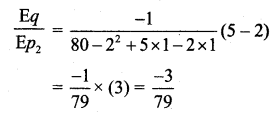Students can download 11th Business Maths Chapter 6 Applications of Differentiation Ex 6.5 Questions and Answers, Notes, Samcheer Kalvi 11th Business Maths Guide Pdf helps you to revise the complete Tamilnadu State Board New Syllabus, helps students complete homework assignments and to score high marks in board exams.

## Tamilnadu Samacheer Kalvi 11th Business Maths Solutions Chapter 6 Applications of Differentiation Ex 6.5

### Samacheer Kalvi 11th Business Maths Applications of Differentiation Ex 6.5 Text Book Back Questions and Answers

Question 1.
Find the marginal productivities of capital (K) and labour (L) if P = 8L – 2K + 3K2 – 2L2 + 7KL when K = 3 and L = 1.
Solution:
P = 8L – 2K + 3K2 – 2L2 + 7KL
Marginal productivity of labour, $$\frac{\partial \mathrm{P}}{\partial \mathrm{L}}$$ = 8 – 0 + 0 – 2(2L) + 7K(1)
= 8 – 4L + 7K
Marginal productivity of labour when K = 3 and L = 1 is
$$\left(\frac{\partial P}{\partial L}\right)_{(3,1)}$$ = 8 – 4 + 21
= 29 – 4
= 25
Marginal productivity of capital, $$\frac{\partial \mathrm{P}}{\partial \mathrm{K}}$$ = 0 – 2(1) + 3(2K) – 0 + 7L(1)
= -2 + 6K + 7L
Marginal productivity of capital when K = 3 and L = 1 is
$$\left(\frac{\partial \mathrm{P}}{\partial \mathrm{K}}\right)_{(3,1)}$$
= -2 + 18 + 7
= -2 + 25
= 23Question 2.
If the production of a firm is given by P = 4LK – L2 + K2, L > 0, K > 0, Prove that L $$\frac{\partial \mathbf{P}}{\partial \mathbf{L}}$$ + K $$\frac{\partial \mathbf{P}}{\partial \mathbf{K}}$$ = 2P.
Solution:
P = 4LK – L2 + K2
P(K, L) = 4LK – L2 + K2
P(tK, tL) = 4(tL) (tK) – t2L2 + t2K2
= t2(4LK – L2 + K2)
= t2P
∴ P is a homogeneous function in L and K of degree 2.
∴ By Euler’s theorem, L $$\frac{\partial \mathbf{P}}{\partial \mathbf{L}}$$ + K $$\frac{\partial \mathbf{P}}{\partial \mathbf{K}}$$ = 2P.

Question 3.
If the production function is z = 3x2 – 4xy + 3y2 where x is the labour and y is the capital, find the marginal productivities of x and y when x = 1, y = 2.
Solution:
Marginal productivity of labour, $$\frac{\partial z}{\partial x}$$ = 6x – 4y
Marginal productivity of labour when x = 1, y = 2 is
$$\left(\frac{\partial z}{\partial x}\right)_{(1,2)}$$ = 6(1) – 4(1)
= 6 – 4
= 2
Marginal productivity of capital, $$\frac{\partial z}{\partial y}$$ = 0 – 4x(1) + 3(2y)
= -4x + 6y
Marginal productivity of qapital when x = 1, y = 2 is
$$\left(\frac{\partial z}{\partial y}\right)_{(1,2)}$$ = -4(1) + 6(2)
= -4 + 12
= 8Question 4.
For the production function P = 3(L)0.4 (K)0.6, find the marginal productivities of labour (L) and capital (K) when L = 10 and K = 6. [use: (0.6)0.6 = 0.736, (1.67)0.4 = 1.2267]
Solution:
Given that P = 3(L)0.4 (K)0.6…….(1)
Differentiating partially with respect to L we get,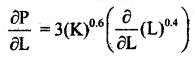When L = 10, k = 6, $$\frac{\partial P}{\partial L}=1.2\left(\frac{6}{10}\right)^{0.6}$$
= 1.2(0.6)0.6
= 1.2(0.736)
i.e., the marginal productivity of labour = 0.8832
Again differentiating partially with respect to ‘k’ we get,
Marginal productivity of labour when L = 10, K = 6 is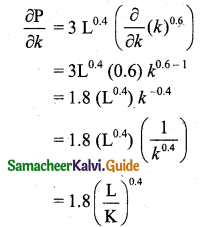Marginal productivity of capital when k = 10, k = 6
= $$1.8\left(\frac{10}{6}\right)^{0.4}$$
= 1.8(1.66666)0.4
= 1.8(1.67)0.4
= 1.8 × 1.2267
= 2.2081

Question 5.
The demand for a quantity A is q = 13 – 2p1 – $$3 p_{2}^{2}$$. Find the partial elasticities $$\frac{\mathbf{E} q}{\mathbf{E} p_{1}}$$ and $$\frac{\mathbf{E q}}{\mathbf{E} p_{2}}$$ when p1 = p2 = 2.
Solution: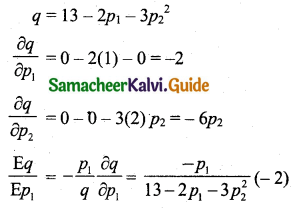When p1 = p2 = 2,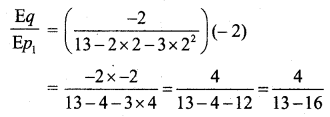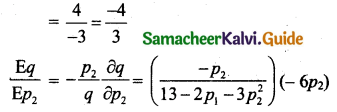When p1 = p2 = 2,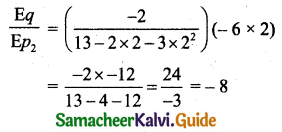Question 6.
The demand for a commodity A is q = 80 – $$p_{1}^{2}$$ + 5p2 – p1p2. Find the partial elasticities $$\frac{\mathbf{E q}}{\mathbf{E} p_{\mathbf{1}}}$$ and $$\frac{\mathbf{E q}}{\mathbf{E} p_{\mathbf{2}}}$$ when p1 = 2, p2 = 1.
Solution: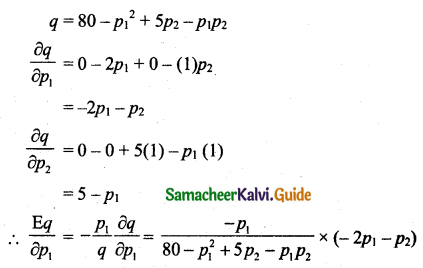When p1 = 2, p2 = 1,When p1 = 2, p2 = 1,